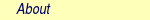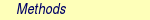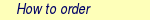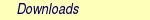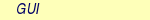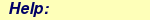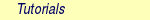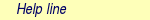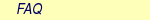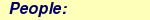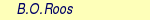MOLCAS manual:Next: 8.31 motra Up: 8. Programs Previous: 8.29 MCPDFT

Subsections

# 8.30 mknemo

The MkNemo module generates intermolecular potential between two subsystems and saves all informations in the NEMO file format.

## 8.30.1 Description

According to the NEMO model of interaction between two subsystems, the MkNemo module splits super-system into two clusters: A and B. The subsystems A and B are defined in the main coordinate system, R. Calculations of the interaction potential between two subsystems are performed for different configurations. At the first step one has to transform both subsystems to the first configuration. An identical transformation to the first configuration is only allowed for one of the subsystems if any coordinates of atoms in the A-subsystem are the same as any atom's coordinates of the B-subsystem. Generally, we define TA and TB as transformation operations of the first and second subsystem to the first configuration(RA,RB) from the main coordinate system (R):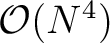Any other configuration can be obtained by transformation ie., translation or rotation of one of the subsystems. For any configuration, one has to calculate the total energy of super-system, A-subsystem with the virtual orbitals of the B-subsystem, and the B-subsystem with virtual orbitals of the A-subsystem at first (unperturbed theory) and second (perturbation) level of theory.

The MkNemo is written in such way that at first step user has to:

1. define different molecules in global coordinate system, R and the molecules can overlay.
2. Define the clusters, A and B, using translation and rotation operations applied for the molecules and for the clusters themselves.
3. Define all possible displacements of any cluster to obtain new configuration.
In the second step user has to provide any input of MOLCAS module which is able to calculate the total energy of the super-system, A-subsystem, and B-subsystem on the first and second level of theory for a given configuration. After any calculation of total energy, one has to call proper block of MkNemo module, GetE, to save energy in the MKNEMO.Conf file. Finally, in the third step, user has to generate new configuration, according to displacement transformations. All three steps are placed in the do-while loop.

## 8.30.2 Files

### 8.30.2.1 Standard input

The MkNemo obeys all rules for format of MOLCAS's input except order of MkNemo's blocks in an input. The input is always preceded by the dummy namelist reference
 &MkNemo &End
and ended by
 End Of Input
.

Example:

&MkNemo &End
.................
End of input

The MkNemo defines transformation as translation,T, or rotation, R, operation in a format:

[ x y z angle]
where the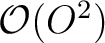is a 3D-vector of translation, or theis a 3D-vector of rotation if the angle parameter is presented, and the angle is an optional parameter which is an angle of rotation around this vector in degrees. Generally, translation and rotation operation do not commute, since that the MkNemo first applys transformation from left to right, i.e.: productmeans that the MkNemo will apply first rotation and then translation.

The input of MkNemo module has been split into four groups of keywords:

• Molecules, Clusters, and Displacement,
• GetEenergy,
• Next,
• Test.
All keywords can be provided in a full name but only first 4 characters ( bold characters) are recognize by MkNemo.

 Keyword Meaning MOLE, CLUS, and DISP The keywords must be provided in right order in the input file. And the blocks of keywords, MOLE, CLUS, and DISP, cannot be split between separated MkNemo inputs. The definition of a Molecule has format: Mole : MoleculeName AtomLabel x y z ......... .. .. .. AtomLabel x y z End where the Mole is keyword which marks beginning of a molecule's block, the MoleculeName is an unique name of molecule, the AtomLabel is the label of atom, and x, y, and z are coordinates of atoms. The name of the molecule is case sensitive, but atom's label is not. In the Cluster's block, user defines a cluster in format: Clus : ClusterName ClusterTransformation MoleculeName MoleculeTransformation ............ ...................... MoleculeName End where the Clus keyword marks beginning of cluster's block, the ClusterName[MoleculeName] is an unique name of cluster[molecule], and the ClusterTransformation[MoleculeTransformation] is an optional argument which defines a transformation of the cluster[molecule]. The cluster and molecule names are case sensitive. The MoleculeName must be defined in a Mole block. The DISPlacement block contains information about transformations of one of the clusters in the format: Disp ClusterName NumberOfSteps Transformation ........... ............. ......... ..... ClusterName NumberOfSteps Transformation End where the ClusterName is a name of one of the clusters which has been defined in Clus block, the NumberOfSteps is a number of steps in which transformation will be reached, the Transformation is a translation or rotation. Any kind of transformations must be provided line by line in the Displacement block and number of transformations is not limited. It means that any row of the DISPlacement block contains information about different transformations. Any new configuration is simply generated from the previous configuration. In this point we can construct final transformation, from the starting configuration to current configuration, as a product of all previous transformations for given subsystem. The MkNemo will store final transformation in orderAny atomic coordinates and vectors of transformations must be provided in a.u. units. The coordinates of transformation vector can be separated by space or a comma .Moreover, the Molecule blocks must be provided first, then the Cluster blocks must appear, and finally Displacement block. In a mixed order, the MkNemo will not be able to recognize a label of molecule[cluster] defined below a block which is using it. An execution of MkNemo module within defined Mole, Clus, and Disp blocks in an input will generate a two coordinate files, named MKNEMO.Axyz and MNEMO.Bxyz. Those files contain coordinates of atoms for clusters B and A respectively, and can be used directly in the SEWARD and GATEWAY (see documentation of GATEWAY for COORD keyword). By default, the Seward or Gateway will apply symmetry, so user must be aware that the displacement transformation can break symmetry of the system and the MkNemo does not control it. If you do not want use symmetry see documentation of Seward or Gateway for details. Example: &MkNemo&End * Molecules definitions Mole : H2o H 1.43 0.0 1.07 H -1.43 0.0 1.07 O 0.00 0.0 0.00 End Mole : Cm3+ Cm 0.0 0.0 0.0 End * Clusters definitions Clus : Cm3+H2o H2o [0.0 0.0 -1.0] [0.0 1.0 0.0 180.0] Cm3+ End Clus : H2O [0.0 0.0 2.0] H2o [0.0 0.0 1.0] End Disp Cm3+H2o : 3 [0.0,0.0,3.0] Cm3+H2o : 1 [0.0,3.0 0.0] H2O : 2 [0.0 0.0,1.0 90.0] End End Of Input In this example, we define two molecules, H2o and Cm3+. Then we define a Cm3+H2o cluster which has been build form H2o and Cm3+ molecule. The H2o molecule has been rotated around Y-axis by the 180 degree and translated along Z-axis by 2 a.u.. The Cm3+ molecule stays unchanged. The second cluster, named H2O has been constructed from translated H2o molecule. The H2o molecule has been translated along Z-axis by 1 a.u.. Then the H2O cluster has been translated along Z-direction by 2 a.u. In the Disp block Cm3+H2o subsystem is translated by vector [0,0,3] in the three steps. Then, in the second row we define translation of H2O cluster by vector [0,3,0] in one step. Finally we rotate H2O cluster by 90 degree around [0,0,1] vector in the two steps. The total number of different configurations is simply a sum of steps: 9=3+1+2+first configuration. GETE The GetEnergy block is used to read total energy stored at RUNFILE, and to save it into the MKNEMO.Conf file. The argument of GetEnergy block must be present and it must be a label from the list below. Use S1 to save the energy of super-system at the first level of theory, S2 to save the energy of super-system at the second level of theory, A1 to save the energy of the A-subsystem with virtual orbitals of B-subsystem at the first level of theory, A2 to save the energy of the A-subsystem with virtual orbitals of B-subsystem at the second level of theory, B1 to save the energy of the B-subsystem with virtual orbitals of A-subsystem at the first level of theory, B2 to save the energy of the B-subsystem with virtual orbitals of A-subsystem at the second level of theory. Please note, that MkNemo does not have any possibility to check what kind of total energy was computed in the previous step by any MOLCAS module. The user has to pay attention on what kind of energy was computed in the previous step. Example: &MkNemo&End GetE A1 End Of Input In this case the total energy which has been computed by a MOLCAS module will be saved as energy of the A-subsystem with virtual orbitals of B-subsystem at the first level of theory. NEXT The Next block is used to save all information about potential curve from previous step into the MKNEMO.Nemo file (the command Next will move data from MKNEMO.Conf file into MKNEMO.Nemo file and will delete MKNEMO.Conf file) and to continue or break an EMIL's loop. This block cannot be used before Mole, Clus, and Disp blocks. Example: &MkNemo&End Next End Of Input TEST The TEST block CAN BE ONLY USED to save verification data for MOLCAS command verify. Example: &MkNemo&End Test End Of Input

Finally the structure of a standard input file for MkNemo module has the following form:

* Loop over configurations

>>>>>>>>>>>>>>>>>>> Do While <<<<<<<<<<<<<<<<<<<<

&MkNemo&End

* Molecules definitions

Mole : MoleculeName
AtomLabel x y z
......... .. .. ..
AtomLabel x y z
End

....................

Mole : MoleculeName
AtomLabel x y z
......... .. .. ..
AtomLabel x y z
End

*
Clus : ClusterName ClusterTransformation
MoleculeName MoleculeTransformation
............ ......................
MoleculeName
MoleculeName
End

Clus : ClusterName ClusterTransformation
MoleculeName MoleculeTransformation
............
MoleculeName
End

Disp
ClusterName NumberOfSteps [x y z alpha]
ClusterName NumberOfSteps [x y z]
........... ............. .............
ClusterName NumberOfSteps [x y z alpha]
End

End Of Input

*************** SUPER-SYSTEM CALCULATION *********************

* Calculation of integrals
&Seward
coord=$Project.MkNemo.Axyz coord=$Project.MkNemo.Bxyz
basis=........
................................

* Energy calculation on the first level of the theory
&Scf
...............................

* Save energy
&MkNemo
GetE=S1

* Energy calculation on the second level of the theory
&MBPT2
...............................

* Save energy
&MkNemo
GetE=S2

*************** A-SUBSYSTEM CALCULATION *********************

* Calculation of integrals
&Seward
coord=$Project.MkNemo.Axyz coord=$Project.MkNemo.Bxyz
* the B-subsytem has charge equal to zero
BSSE=2
basis=........
................................

* Energy calculation on the first level of the theory
&Scf
...............................

* Save energy
&MkNemo&End
GetE=A1

* Energy calculation on the second level of the theory
&MBPT2
...............................

* Save energy
&MkNemo
GetE=A2

*************** B-SUBSYSTEM CALCULATION *********************

* Calculation of integrals
&Seward
coord=$Project.MkNemo.Axyz coord=$Project.MkNemo.Bxyz
* the A-subsytem has charge equal to zero
BSSE=1
basis=........
................................

* Energy calculation on the first level of the theory
&Scf
...............................

* Save energy
&MkNemo
GetE=B1

* Energy calculation on the second level of the theory
&MBPT2
...............................

* Save energy and take next configuration
&MkNemo
GetE=B2; Next

>>>>>>>>>>>>>>>>>>> EndDo <<<<<<<<<<<<<<<<<<<<

Example:

*
* Loop over all configurations
*
>>>>>>>>>>>>>>>>>>> Do While <<<<<<<<<<<<<<<<<<<<

*
* H2O and H2O clusters
*
&MkNemo&End

* Molecules definitions

Mole : H2O
H 1.43 0.0 1.07
H -1.43 0.0 1.07
O 0.00 0.0 0.00
End

* Clusters definitions

Clus : H2O
H2O : [0.0 1.0 0.0 180.0]
End

Clus : h2o [ 0.0 0.0 2.0]
H2O
End

Disp
h2o : 10 [0.0 0.0, 5.0 ]
h2o : 10 [0.0, 0.0, 20.0 ]
h2o : 18 [0.0 0.0 1.0 180.0]
End

End Of Input

*************** SUPER-SYSTEM CALCULATION *********************

* Calculation of integrals

&Seward
NEMO
Title=Sypersystem
Douglas-Kroll
ANGM= 0.0 0.0 0.0; AMFI
COORD=$Project.MkNemo.Axyz;Coord=$Project.MkNemo.Bxyz
basis=H.ano-rcc...2s1p.,O.ano-rcc.Roos..4s3p2d1f.

* Energy calculation on the first level of the theory
&Scf
Title=Supersystem; Occupied=10; Iterations=30; Disk=1 0

* Save energy
&MkNemo
GetE=S1

* Energy calculation on the second level of the theory
&MBPT2
Title=Sypersystem; Threshold=1.0d-14 1.0d-14 1.0d-14

* Save energy
&MkNemo
GetE=S2

*************** A-SUBSYSTEM CALCULATION *********************

* Calculation of integrals

&Seward
NEMO
Title=A-system
Douglas-Kroll
ANGM= 0.0 0.0 0.0; AMFI
COORD=$Project.MkNemo.Axyz;Coord=$Project.MkNemo.Bxyz
basis=H.ano-rcc...2s1p.,O.ano-rcc.Roos..4s3p2d1f.
BSSE=2

* Energy calculation on the first level of the theory
&Scf
Title=A-subsystem; Occupied=5; Iterations=30; Disk=1 0

* Save energy
&MkNemo
GetE=A1

* Energy calculation on the second level of the theory
&MBPT2
Title=A-subsystem; Threshold=1.0d-14 1.0d-14 1.0d-14

* Save energy
&MkNemo
GetE=A2

*************** B-SUBSYSTEM CALCULATION *********************

* Calculation of integrals

&Seward
NEMO
Title=A-system
Douglas-Kroll
ANGM= 0.0 0.0 0.0; AMFI
COORD=$Project.MkNemo.Axyz;Coord=$Project.MkNemo.Bxyz
basis=H.ano-rcc...2s1p.,O.ano-rcc.Roos..4s3p2d1f.
BSSE=1

* Energy calculation on the first level of the theory

&Scf
Title=B-subsystem; Occupied=5; Iterations=30; Disk=1 0

* Save energy
&MkNemo
GetE=B1

* Energy calculation on the second level of the theory
&MBPT2
Title=B-subsytem; Threshold= 1.0d-14 1.0d-14 1.0d-14

* Save energy and take next configuration
&MkNemo
GetE=B2; Next

>>>>>>>>>>>>>>>>>>> EndDo <<<<<<<<<<<<<<<<<<<<

In this example we calculate potential energy curve for interaction between two water clusters. The A-cluster, H2O, was rotated around Y-axis about 180 degrees. The B-subsystem, h2o,has been translated along Z-axis by 2 a.u.. In the Disp block we have defined 20 translation operations for h2o cluster and 18 rotation operations for H2O cluster. For energy calculations of super-system, A-subsystem, and B-subsystem, at first level of theory we used SCF module, and MBPT2 at second level of theory, respectively. After a calculation of energy we save calculated results using keyword GetE with proper argument in the MKNEMO.Conf file of MkNemo module. Finally, by calling block Next of MkNemo, we save all informations about potential for given configuration and we generate new configuration. This procedure will be repeated for all translations and rotations defined in the Displacement block.

### 8.30.2.2 Input files

Apart from the standard input unit MkNemo will use the following input files.

FileContents
MKNEMO.InputA MkNemo's input file contains the latest preprocessed input.
MKNEMO.RestartThe MKNEMO.Restart is a restart file, which will be generated by MkNemo at the first run if the file does not exist. Any call of group of command: Mole, Clus, and Disp will be updated and the restart file is saved in user's $CurrDir. If MkNemo calculation crashes, one can fix a reason of crash, copy restart and MKNEMO.Nemo files to$WorkDir, and run the calculation again. The MkNemo will restart calculation from the last point which has been finished successfully. If the MKNEMO.Nemo file will not be copied the MkNemo will generate a new one and will overwrite the file in your \$CurrDirr if any exist. Beware of it.

The restart file is formated :

<Restart> RowInDisp Step'sNum LoopControl</Restart>
where the RowInDisp is the index of currently used row in the Disp block and the Step'sNum is the current number of step for a given displacement's row. Ex. If a displacement row, RowInDisp, is 3, which corresponds to a displacement row, H2O 4 [0,4,0], and Step'sNum is equal to 2 then it means that current displacement vector is [0,2,0]. The LoopControl parameter is a return code. The command Next will read this value and use it to continue looping or breaking a loop.

MKNEMO.ConfThe MKNEMO.Conf is a file which stores block Mole, Clus, and Eneries in similar format like it is define in the input of theMkNemo, but within XML format. The propose of this file is to share definition of molecules, clusters, and energies between different blocks of namelist,
 &MkNemo
. A format of this file is:

* Configuration definition - contains informations
<Configuration>

* Definition of molecule
<Molecule Name=''Name of molecule''>
labelOfAtom x y z
........... .. .. ..
labelOfAtom x y z
</Molecule>

....................................

<Molecule Name=''Name of molecule''>
labelOfAtom x y z
........... .. .. ..
labelOfAtom x y z
</Molecule>

* Definition of cluster
<Cluster Name=''Name of cluster A'' Transformation=''x y z q0 q1 q2 q3''>
labelOfMolecule x' y' z' q0' q1' q2' a3'
............... .. .. .. .. .. .. ..
labelOfMolecule x' y' z' q0' q1' q2' a3'
</Cluster>

<Cluster Name=''Name of cluster B'' Transformation=''x y z q0 q1 q2 q3''>
labelOfMolecule x' y' z' q0' q1' q2' a3'
............... .. .. .. .. .. .. ..
labelOfMolecule x' y' z' q0' q1' q2' a3'
</Cluster>

* Enerigies definition
<Energies>
EnegyLabel MethodLabel Energy
.......... ........... ......
EnegyLabel MethodLabel Energy
</Energies>
</Configuration>
where the EnergyLabel is one of labels defined in the GetE block, the MethodLabel is a name of method which has been used to calculate energy, and Energy is a vector of eigenvalues. The Next command will save energy information into a MKNEMO.Nemo file, and will clear this file. Hacking hint: If you want to use Rassi then do not use call of command GetE but postprocess output and print eigenvalues to the MKNEMO.Conf file in the right format (use ! in user input to execute shell command for postprocessing of output)

Files of the SEWARD, SCF, RASSCF, MBPT2, MOTRA, CCSDT, and CASPT2 modules are needed to get total energy on each level of theory for subsystems and super-system.

### 8.30.2.3 Output files

In addition to the standard output unit MkNemo will generate the following files.

 File Contents MKNEMO.Axyz,MKNEMO.Bxyz The MKNEMO.*xyz file is a file of coordinates in format: NumberOfAtoms AdditionalLine AtomLabel x y z ......... .. .. .. AtomLabel x y z where the NumberOfAtoms is a number of atoms in the file, the AdditionalLine is a line where one can provide unit of coordinate (currently MkNemo supports only a.u.), the AtomLabel is a label of atom, and x, y, z is a vector of coordinates. RUNFILE A file with informations needed by the block of MOLCAS. MKNEMO.Nemo On this file MkNemo will store all information about intermolecular potential in the NEMO file format. This format is used by NEMO to fit intermolecular potential to the NEMO model. The format of this file is defined as follows: * Definition of configuration ............................. ............................. ............................. Where configuration block is defined like in the MKNEMO.Conf.

## 8.30.3 Dependencies

The MkNemo depends on the modules of MOLCAS program, which calculate the total energy of the system.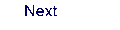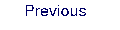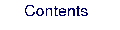Next: 8.31 motra Up: 8. Programs Previous: 8.29 MCPDFT